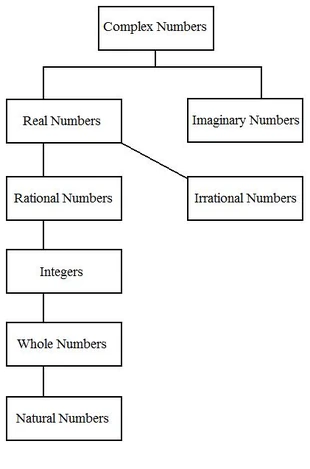38 PagesA simplified "tree" of number systems.

In algebra, imaginary numbers are part of the complex number system in which real numbers cannot measure.

The symbol for imaginary numbers is i. You can define what i is equivalent to, but you cannot measure it in real terms.

i is equal to the square root of -1.This means that i2 is equal to -1.Therefore, i3 is equal to -i, or the negative square root of -1.Hence, i4 is equal to 1.This pattern of i, -1, -i, and 1 continues ad infinitum in the same exact manner shown.

## Simplyfing In Terms of i

With i, you can simplify the square roots of negative numbers.## Defining Complex Numbers

All complex numbers have a real part and an imaginary part. This can be shown as follows:, where a is real and b is imaginary.

Below are several examples of complex numbers.,,,,, and evenare all complex numbers.

## Multiplying Complex Numbers

Multiplying complex numbers is not that much different than multiplying algebraic expressions. I'll show you how so below.

1. Copy the problem...2. LOIF it (Last - Outside - Inside - First), which is like FOIL in reverse...3. Combine like terms...WATCH OUT! You're not done! Remember, i2 = -1.4. Simplify the exponents...5. And combine the like terms again for the final answer.Community content is available under CC-BY-SA unless otherwise noted.